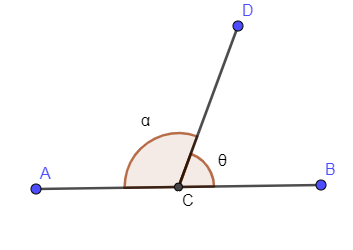What is the supplementary angle of ${{40}^{\circ }}$?Verified
150k+ views
Hint: We know that supplementary of any angle is the subtraction of that angle from ${{180}^{\circ }}$. So, in this problem we have given an angle of ${{40}^{\circ }}$so its supplementary angle is calculated by subtracting ${{40}^{\circ }}$from ${{180}^{\circ }}$. The result of this subtraction is the supplementary angle that we are looking for.

In the above problem, we are asked to find the supplementary angle of ${{40}^{\circ }}$.
The supplementary angle of any angle is equal to the subtraction of that angle from ${{180}^{\circ }}$.
Let us assume angle $\theta$ is the angle with respect to which we have to find the supplementary angle and the supplementary angle be $\alpha$.
In the below diagram, we have shown a straight line along with the angle $\theta$ and angle $\alpha$.As you can see that $\theta \And \alpha$ are forming the linear pairs so the summation of both these angles is equal to ${{180}^{\circ }}$.
Adding $\theta \And \alpha$ and equating it to ${{180}^{\circ }}$ we get,
\begin{align} & \theta +\alpha ={{180}^{\circ }} \\ & \Rightarrow \alpha ={{180}^{\circ }}-\theta ......Eq.(1) \\ \end{align}
Now, we have assumed that $\alpha$ is the supplementary angle of $\theta$.
In the above question, we are asked to find the supplementary angle of ${{40}^{\circ }}$ so from the above description of supplementary angle, $\theta ={{40}^{\circ }}$ so substituting this value of $\theta$ in eq. (1) we will get the value of supplementary angle corresponding to ${{40}^{\circ }}$.
\begin{align} & \alpha ={{180}^{\circ }}-\theta \\ & \Rightarrow \alpha ={{180}^{\circ }}-{{40}^{\circ }} \\ & \Rightarrow \alpha ={{140}^{\circ }} \\ \end{align}

Hence, we have found the supplementary angle of ${{40}^{\circ }}$ as ${{140}^{\circ }}$.

Note: You can cross check the value of supplementary angle of ${{40}^{\circ }}$ that we have calculated above by using the relation that sum of the angle and its supplement is ${{180}^{\circ }}$.
The angle is ${{40}^{\circ }}$ and its supplementary that we have calculated above is ${{140}^{\circ }}$. Now, the addition of these two angles must be equal to ${{180}^{\circ }}$.
${{40}^{\circ }}+{{140}^{\circ }}={{180}^{\circ }}$
Adding the left hand side of the above equation we get,
${{180}^{\circ }}={{180}^{\circ }}$
As you can see that L.H.S = R.H.S so the supplementary angle that we have calculated above is correct.